Скачать презентацию OPTIONS MARKETS Options Similar to futures

8178841fdc310095e1e892036451d88c.ppt

• Количество слайдов: 56OPTIONS MARKETS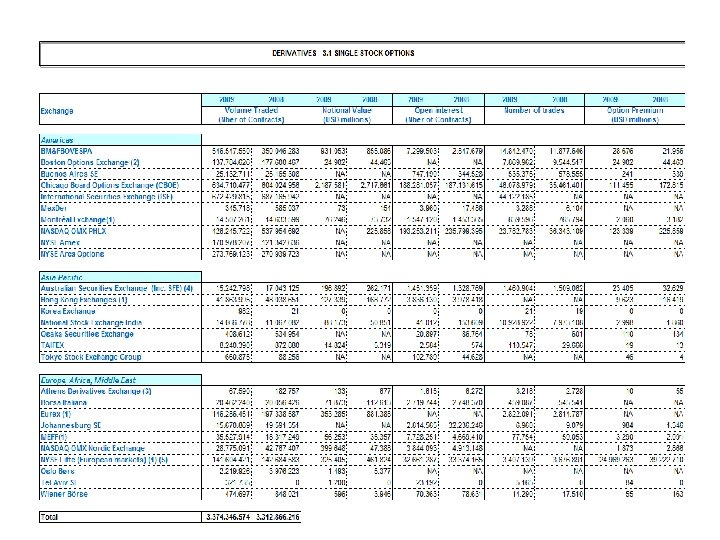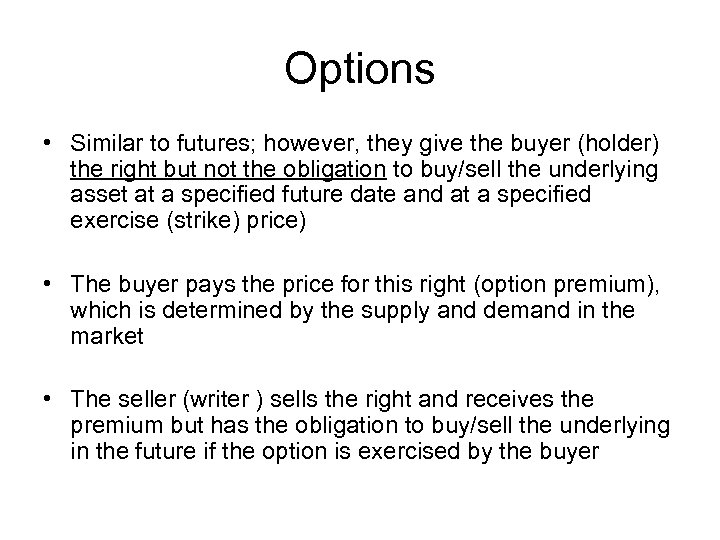Options • Similar to futures; however, they give the buyer (holder) the right but not the obligation to buy/sell the underlying asset at a specified future date and at a specified exercise (strike) price) • The buyer pays the price for this right (option premium), which is determined by the supply and demand in the market • The seller (writer ) sells the right and receives the premium but has the obligation to buy/sell the underlying in the future if the option is exercised by the buyer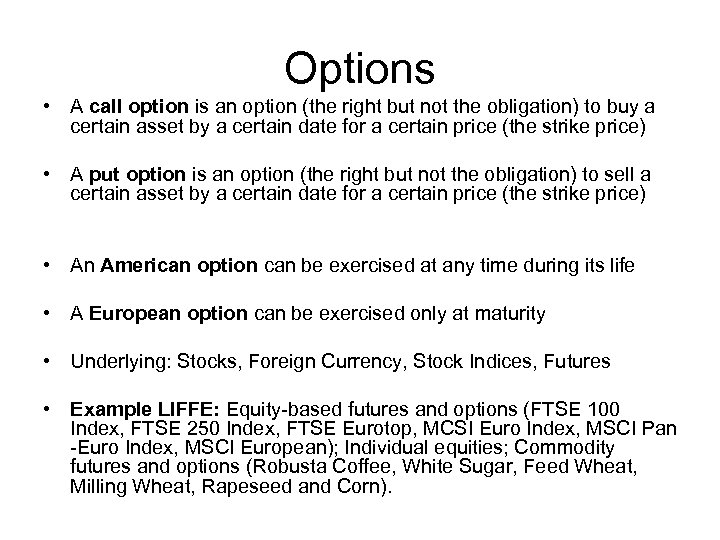Options • A call option is an option (the right but not the obligation) to buy a certain asset by a certain date for a certain price (the strike price) • A put option is an option (the right but not the obligation) to sell a certain asset by a certain date for a certain price (the strike price) • An American option can be exercised at any time during its life • A European option can be exercised only at maturity • Underlying: Stocks, Foreign Currency, Stock Indices, Futures • Example LIFFE: Equity-based futures and options (FTSE 100 Index, FTSE 250 Index, FTSE Eurotop, MCSI Euro Index, MSCI Pan -Euro Index, MSCI European); Individual equities; Commodity futures and options (Robusta Coffee, White Sugar, Feed Wheat, Milling Wheat, Rapeseed and Corn).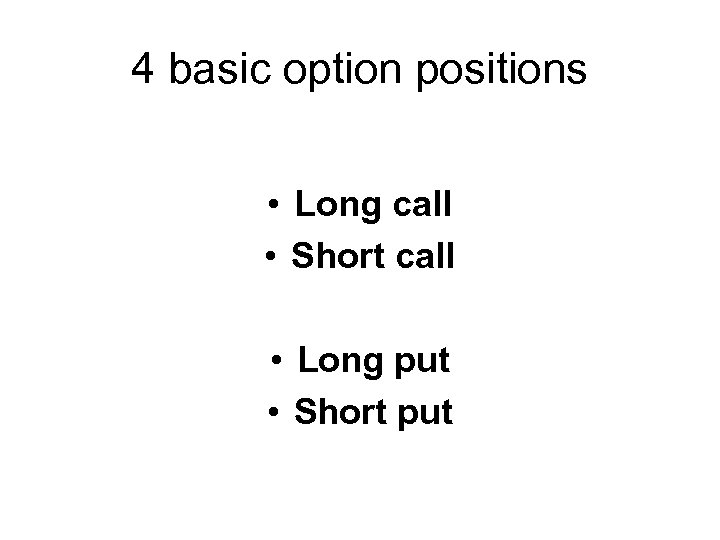4 basic option positions • Long call • Short call • Long put • Short put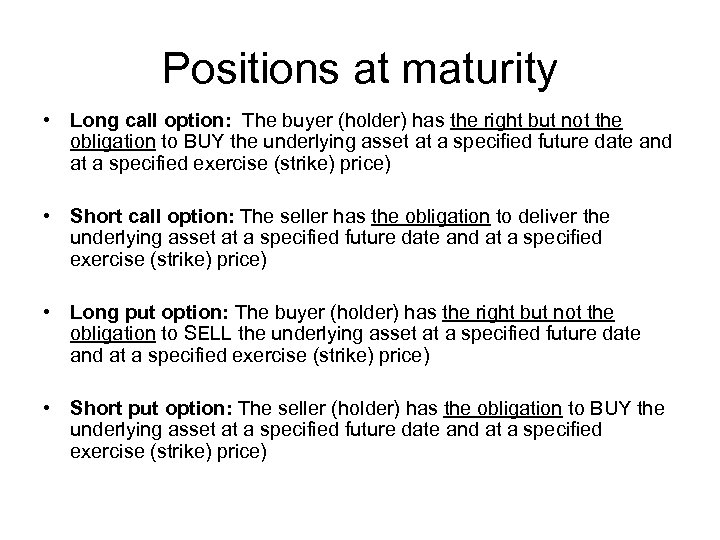Positions at maturity • Long call option: The buyer (holder) has the right but not the obligation to BUY the underlying asset at a specified future date and at a specified exercise (strike) price) • Short call option: The seller has the obligation to deliver the underlying asset at a specified future date and at a specified exercise (strike) price) • Long put option: The buyer (holder) has the right but not the obligation to SELL the underlying asset at a specified future date and at a specified exercise (strike) price) • Short put option: The seller (holder) has the obligation to BUY the underlying asset at a specified future date and at a specified exercise (strike) price)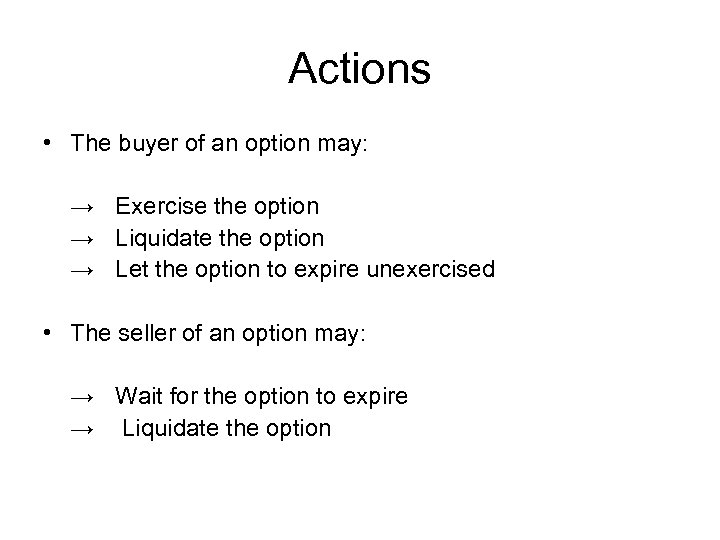Actions • The buyer of an option may: → Exercise the option → Liquidate the option → Let the option to expire unexercised • The seller of an option may: → Wait for the option to expire → Liquidate the option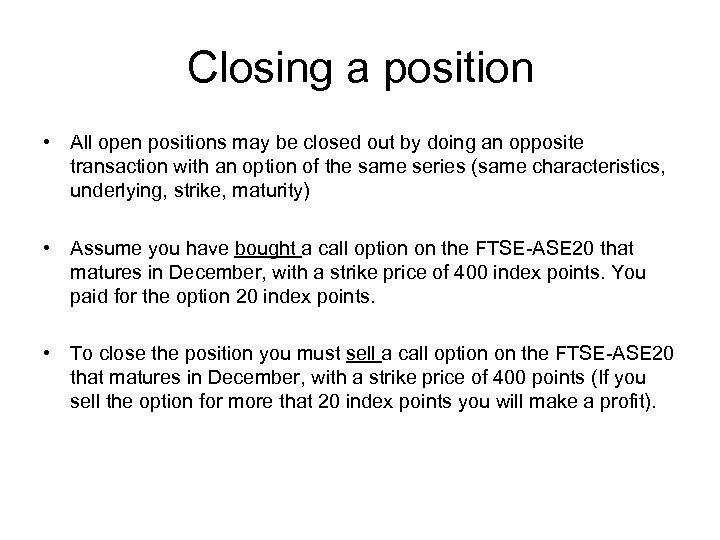Closing a position • All open positions may be closed out by doing an opposite transaction with an option of the same series (same characteristics, underlying, strike, maturity) • Assume you have bought a call option on the FTSE-ASE 20 that matures in December, with a strike price of 400 index points. You paid for the option 20 index points. • To close the position you must sell a call option on the FTSE-ASE 20 that matures in December, with a strike price of 400 points (If you sell the option for more that 20 index points you will make a profit).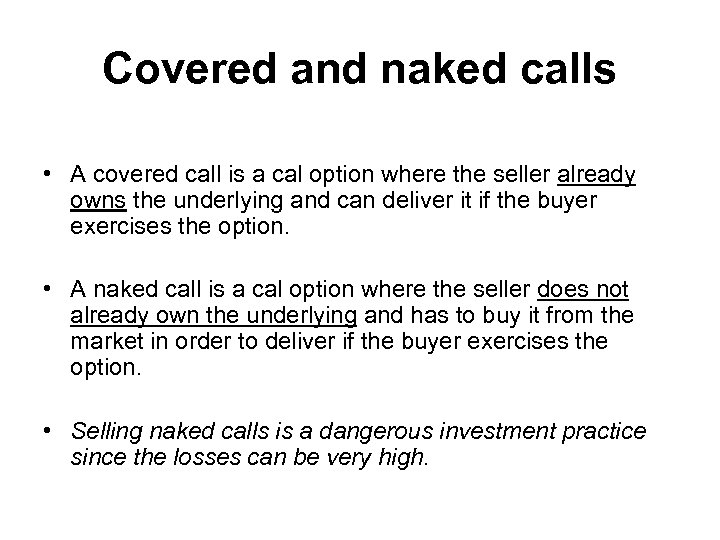Covered and naked calls • A covered call is a cal option where the seller already owns the underlying and can deliver it if the buyer exercises the option. • A naked call is a cal option where the seller does not already own the underlying and has to buy it from the market in order to deliver if the buyer exercises the option. • Selling naked calls is a dangerous investment practice since the losses can be very high.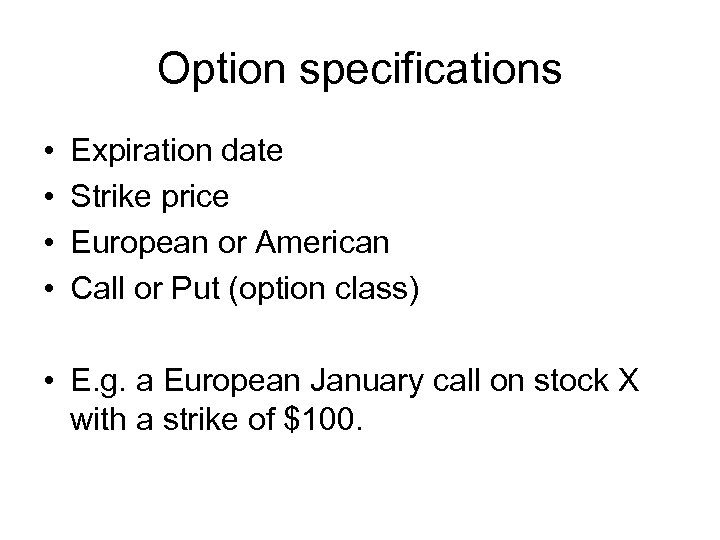Option specifications • • Expiration date Strike price European or American Call or Put (option class) • E. g. a European January call on stock X with a strike of \$100.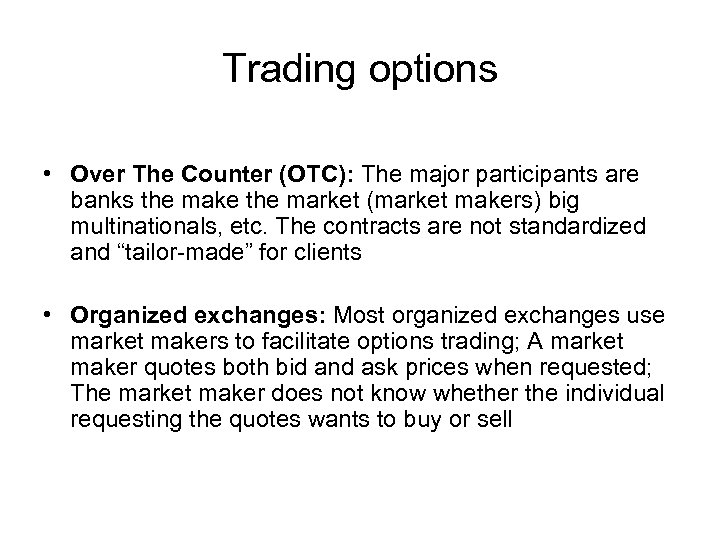Trading options • Over The Counter (OTC): The major participants are banks the make the market (market makers) big multinationals, etc. The contracts are not standardized and “tailor-made” for clients • Organized exchanges: Most organized exchanges use market makers to facilitate options trading; A market maker quotes both bid and ask prices when requested; The market maker does not know whether the individual requesting the quotes wants to buy or sell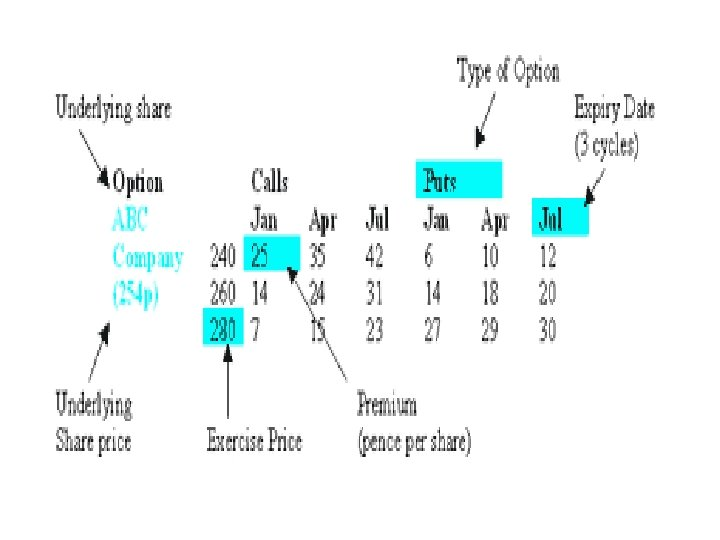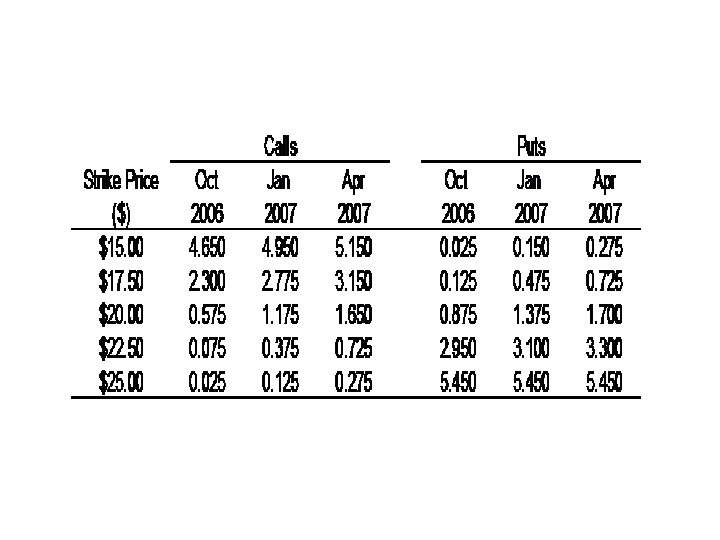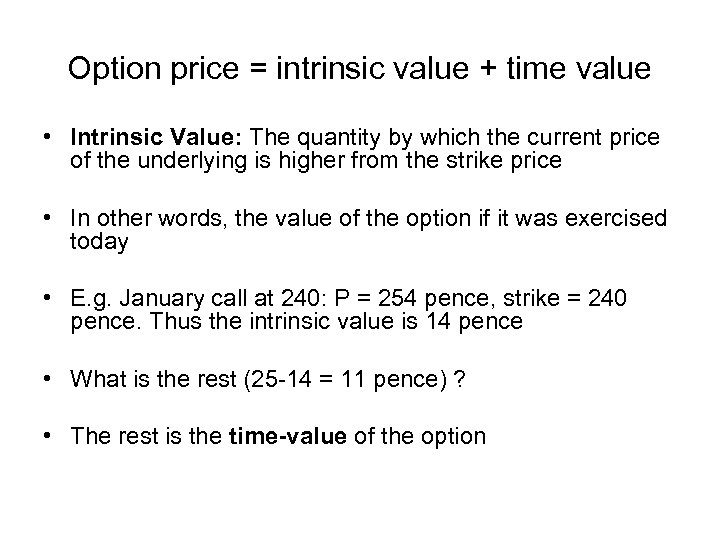Option price = intrinsic value + time value • Intrinsic Value: The quantity by which the current price of the underlying is higher from the strike price • In other words, the value of the option if it was exercised today • E. g. January call at 240: P = 254 pence, strike = 240 pence. Thus the intrinsic value is 14 pence • What is the rest (25 -14 = 11 pence) ? • The rest is the time-value of the option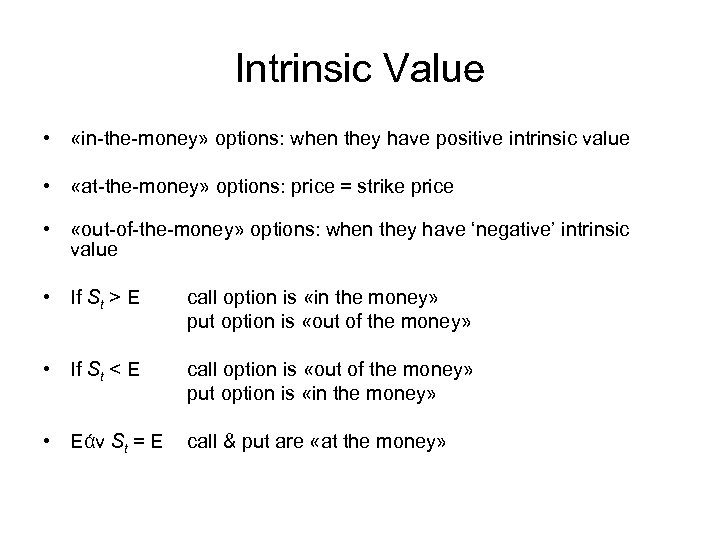Intrinsic Value • «in-the-money» options: when they have positive intrinsic value • «at-the-money» options: price = strike price • «out-of-the-money» options: when they have ‘negative’ intrinsic value • If St > E call option is «in the money» put option is «out of the money» • If St < E call option is «out of the money» put option is «in the money» • Εάν St = E call & put are «at the money»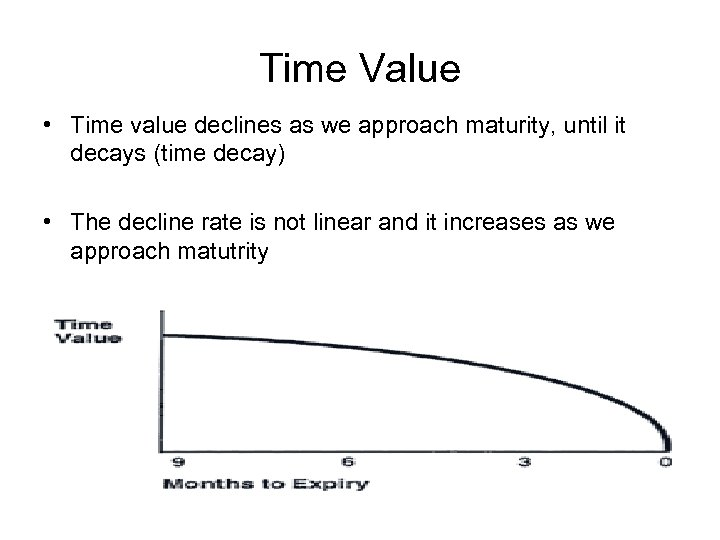Time Value • Time value declines as we approach maturity, until it decays (time decay) • The decline rate is not linear and it increases as we approach matutrity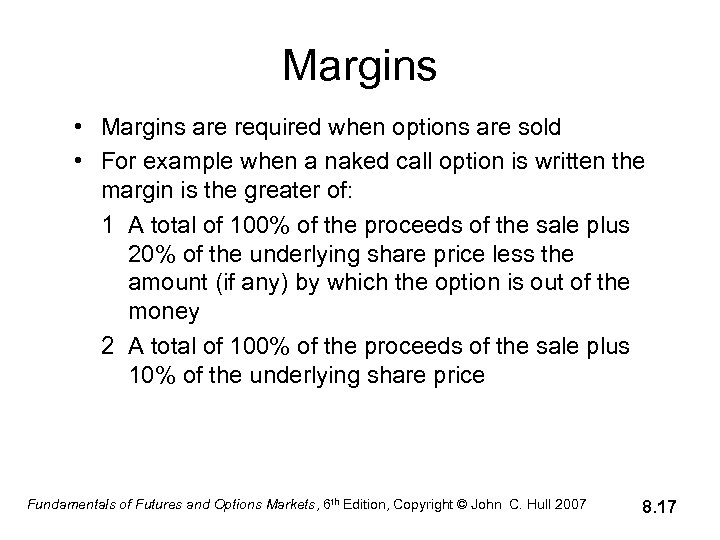Margins • Margins are required when options are sold • For example when a naked call option is written the margin is the greater of: 1 A total of 100% of the proceeds of the sale plus 20% of the underlying share price less the amount (if any) by which the option is out of the money 2 A total of 100% of the proceeds of the sale plus 10% of the underlying share price Fundamentals of Futures and Options Markets, 6 th Edition, Copyright © John C. Hull 2007 8. 17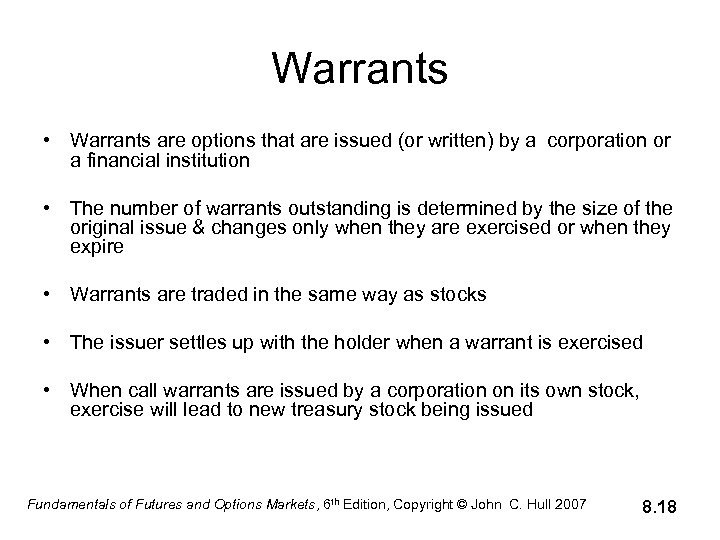Warrants • Warrants are options that are issued (or written) by a corporation or a financial institution • The number of warrants outstanding is determined by the size of the original issue & changes only when they are exercised or when they expire • Warrants are traded in the same way as stocks • The issuer settles up with the holder when a warrant is exercised • When call warrants are issued by a corporation on its own stock, exercise will lead to new treasury stock being issued Fundamentals of Futures and Options Markets, 6 th Edition, Copyright © John C. Hull 2007 8. 18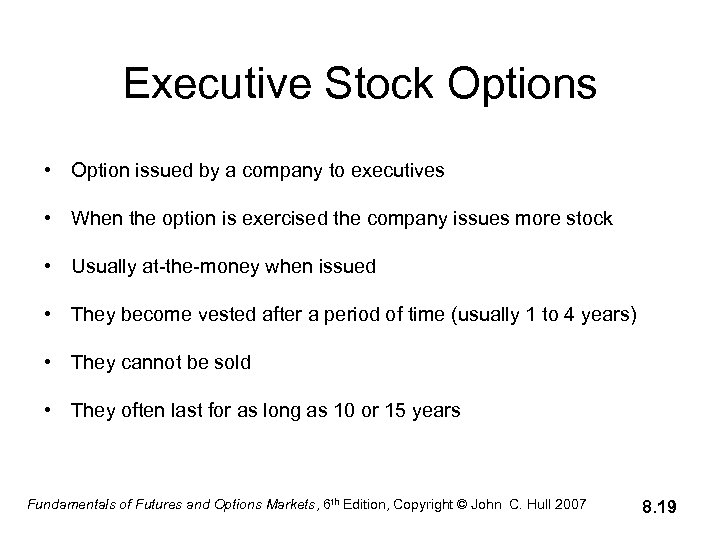Executive Stock Options • Option issued by a company to executives • When the option is exercised the company issues more stock • Usually at-the-money when issued • They become vested after a period of time (usually 1 to 4 years) • They cannot be sold • They often last for as long as 10 or 15 years Fundamentals of Futures and Options Markets, 6 th Edition, Copyright © John C. Hull 2007 8. 19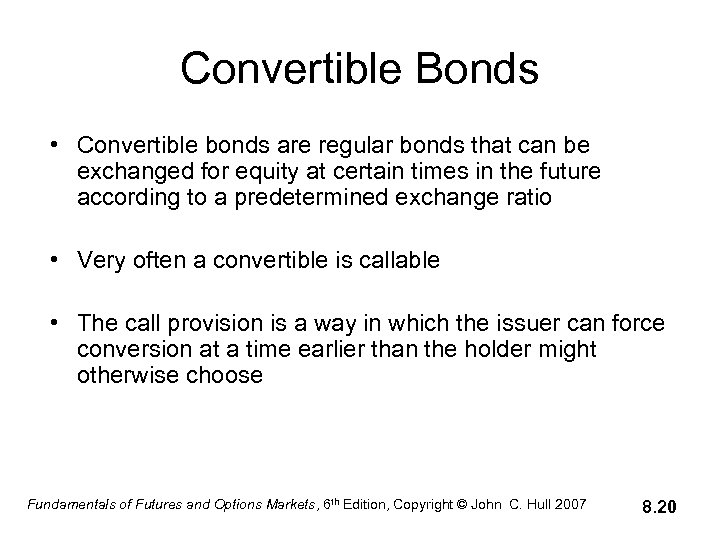Convertible Bonds • Convertible bonds are regular bonds that can be exchanged for equity at certain times in the future according to a predetermined exchange ratio • Very often a convertible is callable • The call provision is a way in which the issuer can force conversion at a time earlier than the holder might otherwise choose Fundamentals of Futures and Options Markets, 6 th Edition, Copyright © John C. Hull 2007 8. 20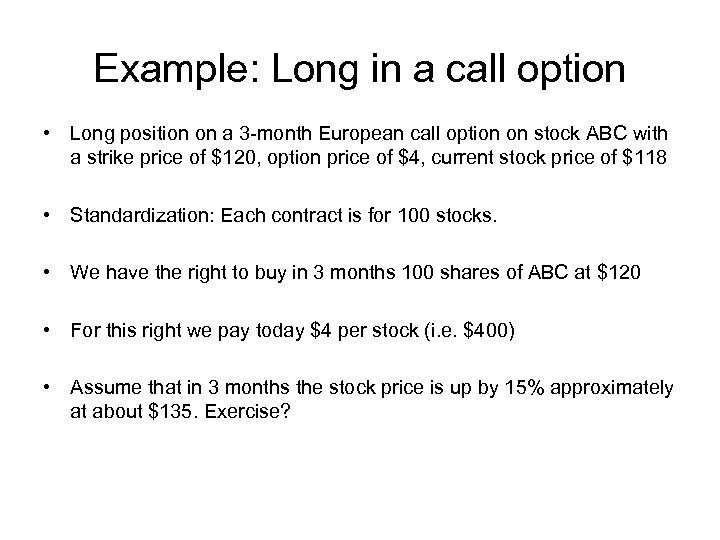Example: Long in a call option • Long position on a 3 -month European call option on stock ABC with a strike price of \$120, option price of \$4, current stock price of \$118 • Standardization: Each contract is for 100 stocks. • We have the right to buy in 3 months 100 shares of ABC at \$120 • For this right we pay today \$4 per stock (i. e. \$400) • Assume that in 3 months the stock price is up by 15% approximately at about \$135. Exercise?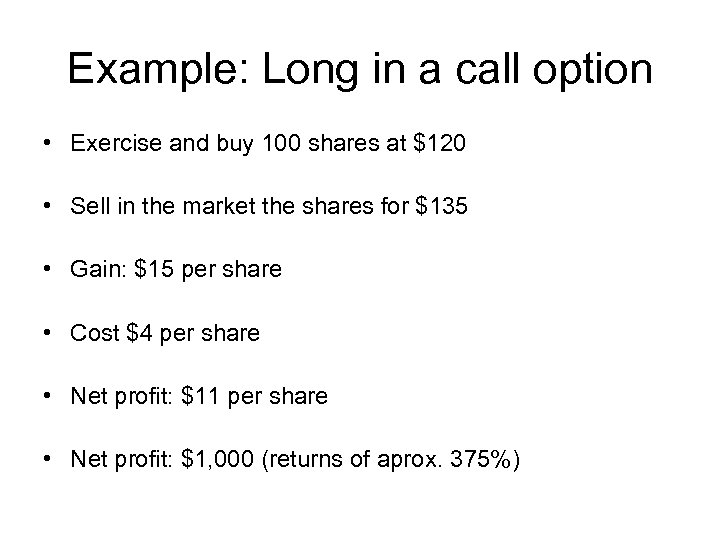Example: Long in a call option • Exercise and buy 100 shares at \$120 • Sell in the market the shares for \$135 • Gain: \$15 per share • Cost \$4 per share • Net profit: \$11 per share • Net profit: \$1, 000 (returns of aprox. 375%)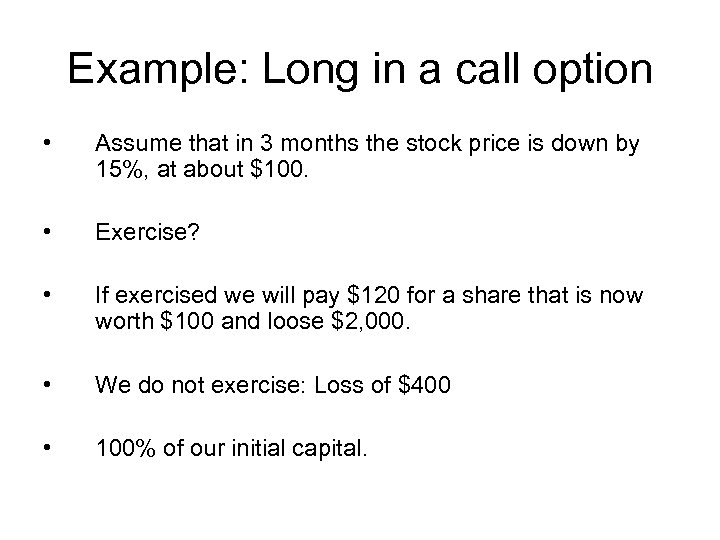Example: Long in a call option • Assume that in 3 months the stock price is down by 15%, at about \$100. • Exercise? • If exercised we will pay \$120 for a share that is now worth \$100 and loose \$2, 000. • We do not exercise: Loss of \$400 • 100% of our initial capital.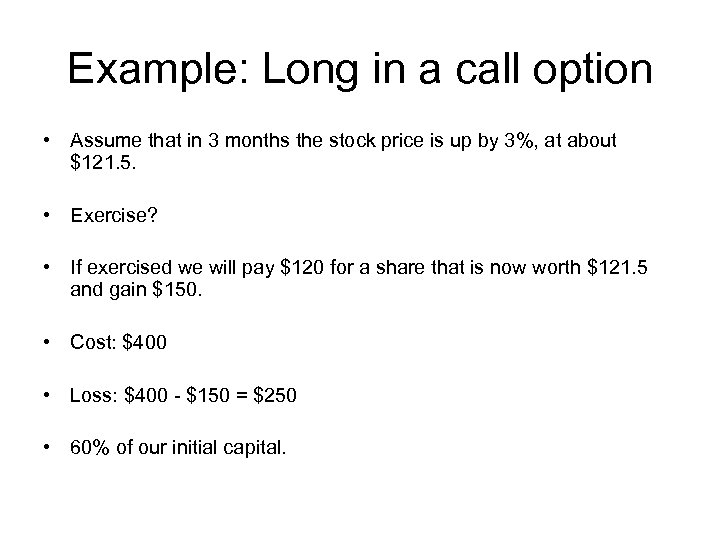Example: Long in a call option • Assume that in 3 months the stock price is up by 3%, at about \$121. 5. • Exercise? • If exercised we will pay \$120 for a share that is now worth \$121. 5 and gain \$150. • Cost: \$400 • Loss: \$400 - \$150 = \$250 • 60% of our initial capital.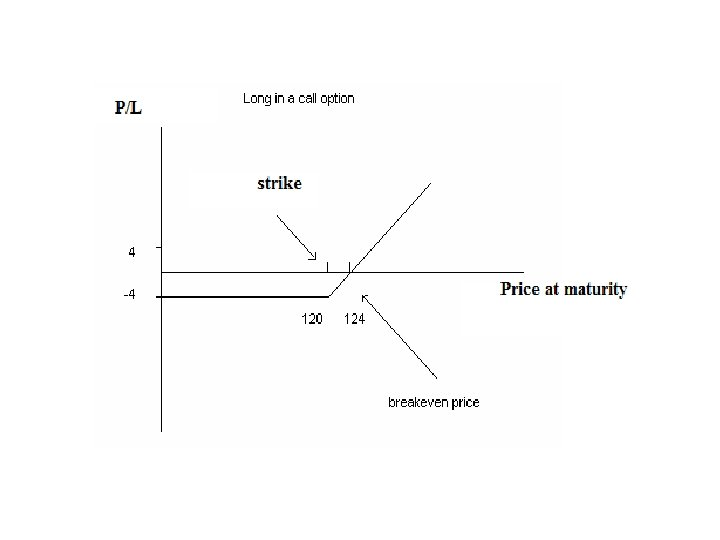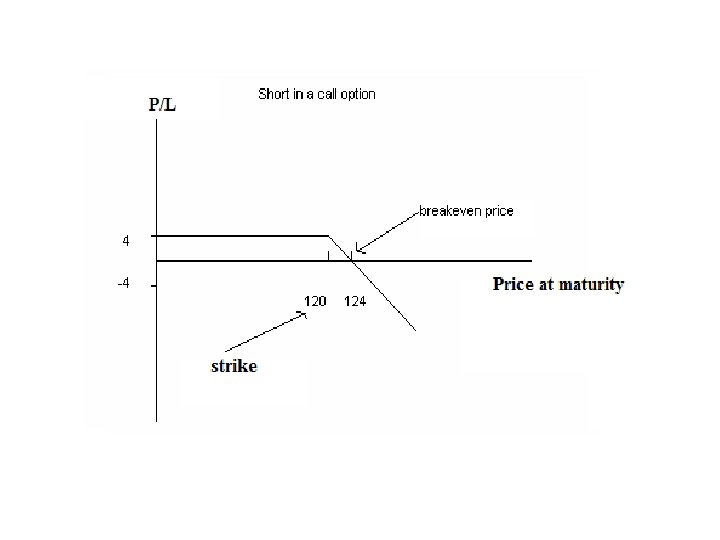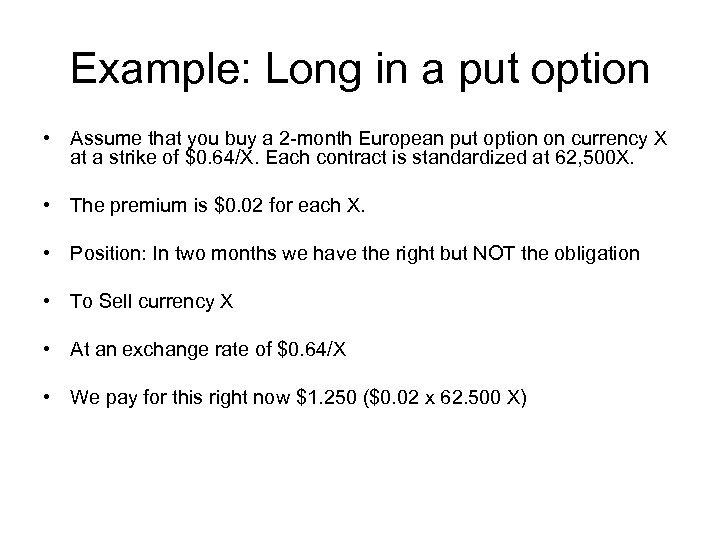Example: Long in a put option • Assume that you buy a 2 -month European put option on currency Χ at a strike of \$0. 64/Χ. Each contract is standardized at 62, 500Χ. • The premium is \$0. 02 for each X. • Position: In two months we have the right but NOT the obligation • To Sell currency X • At an exchange rate of \$0. 64/Χ • We pay for this right now \$1. 250 (\$0. 02 x 62. 500 Χ)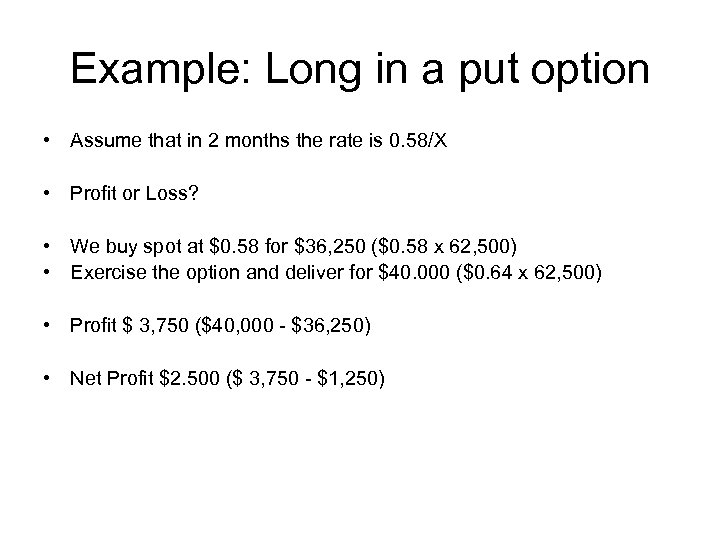Example: Long in a put option • Assume that in 2 months the rate is 0. 58/Χ • Profit or Loss? • We buy spot at \$0. 58 for \$36, 250 (\$0. 58 x 62, 500) • Exercise the option and deliver for \$40. 000 (\$0. 64 x 62, 500) • Profit \$ 3, 750 (\$40, 000 - \$36, 250) • Net Profit \$2. 500 (\$ 3, 750 - \$1, 250)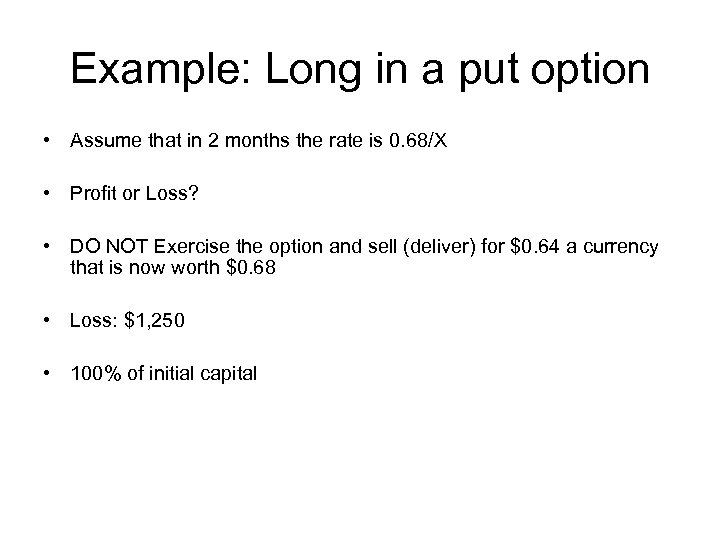Example: Long in a put option • Assume that in 2 months the rate is 0. 68/Χ • Profit or Loss? • DO NOT Exercise the option and sell (deliver) for \$0. 64 a currency that is now worth \$0. 68 • Loss: \$1, 250 • 100% of initial capital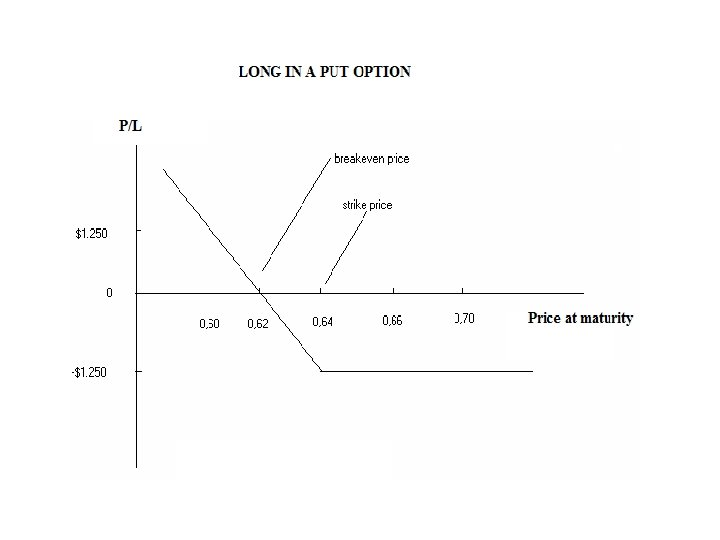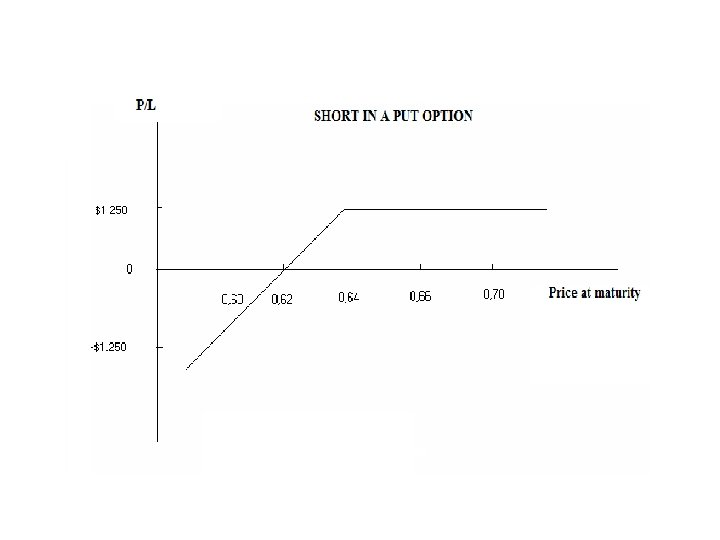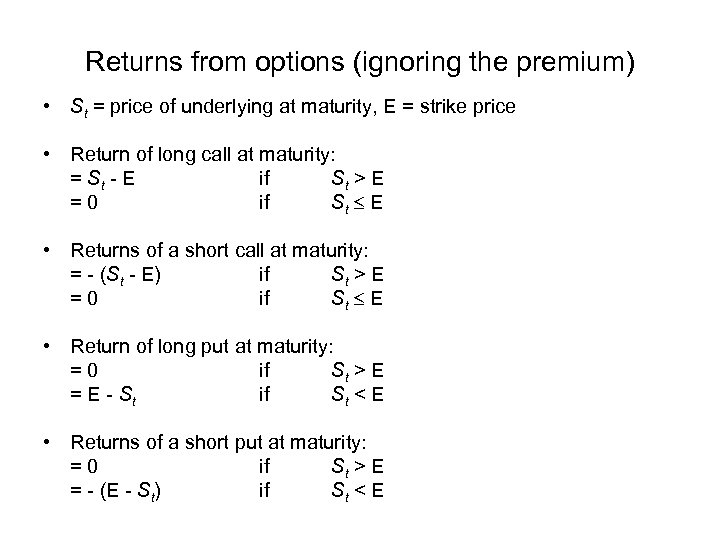Returns from options (ignoring the premium) • St = price of underlying at maturity, E = strike price • Return of long call at maturity: = St - E if St > E =0 if St E • Returns of a short call at maturity: = - (St - E) if St > E =0 if St E • Return of long put at maturity: =0 if St > E = E - St if St < E • Returns of a short put at maturity: =0 if St > E = - (E - St) if St < E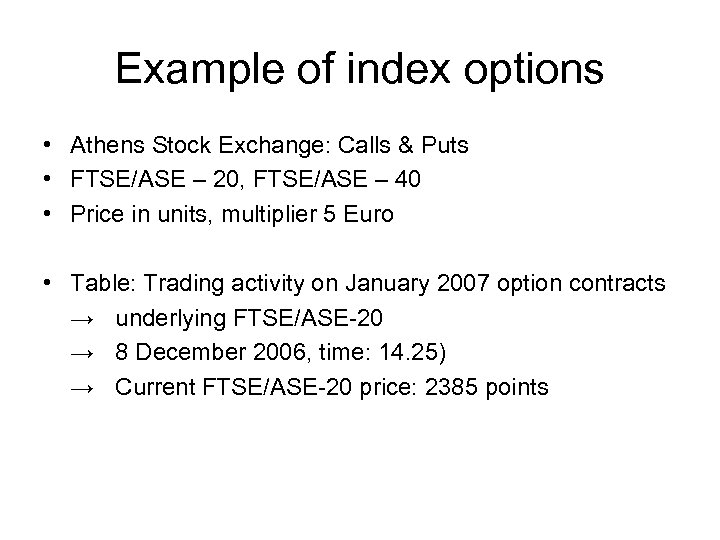Example of index options • Athens Stock Exchange: Calls & Puts • FTSE/ASE – 20, FTSE/ASE – 40 • Price in units, multiplier 5 Euro • Table: Trading activity on January 2007 option contracts → underlying FTSE/ASE-20 → 8 December 2006, time: 14. 25) → Current FTSE/ASE-20 price: 2385 points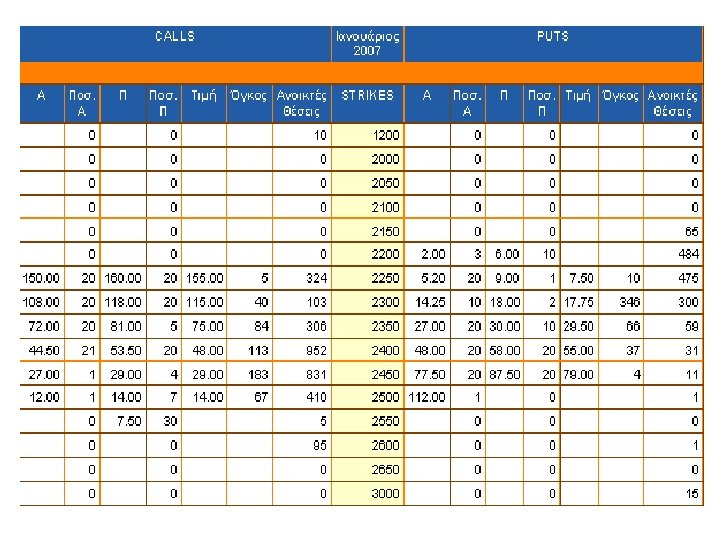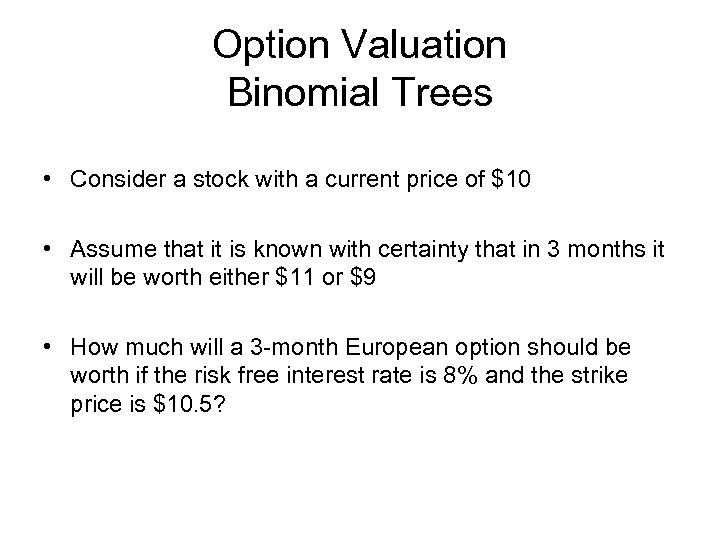Option Valuation Binomial Trees • Consider a stock with a current price of \$10 • Assume that it is known with certainty that in 3 months it will be worth either \$11 or \$9 • How much will a 3 -month European option should be worth if the risk free interest rate is 8% and the strike price is \$10. 5?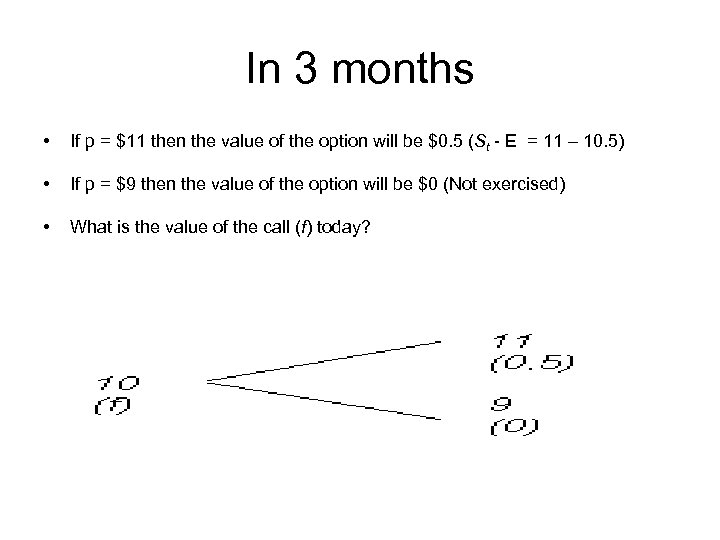In 3 months • If p = \$11 then the value of the option will be \$0. 5 (St - E = 11 – 10. 5) • If p = \$9 then the value of the option will be \$0 (Not exercised) • What is the value of the call (f) today?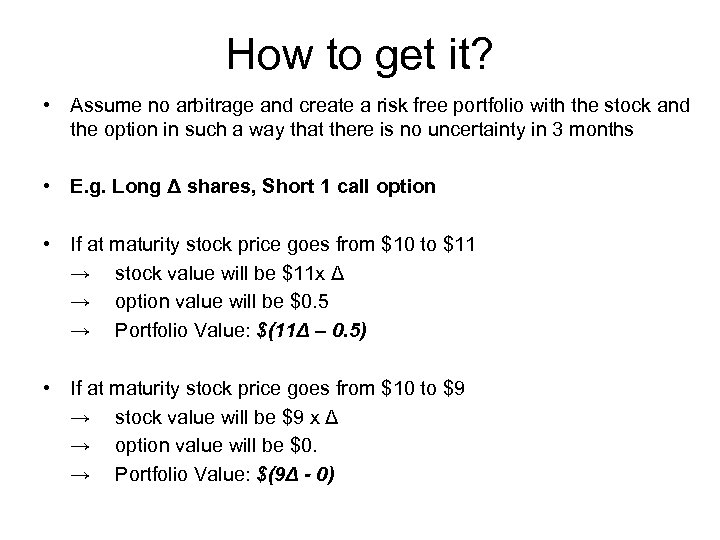How to get it? • Assume no arbitrage and create a risk free portfolio with the stock and the option in such a way that there is no uncertainty in 3 months • E. g. Long Δ shares, Short 1 call option • If at maturity stock price goes from \$10 to \$11 → stock value will be \$11 x Δ → option value will be \$0. 5 → Portfolio Value: \$(11Δ – 0. 5) • If at maturity stock price goes from \$10 to \$9 → stock value will be \$9 x Δ → option value will be \$0. → Portfolio Value: \$(9Δ - 0)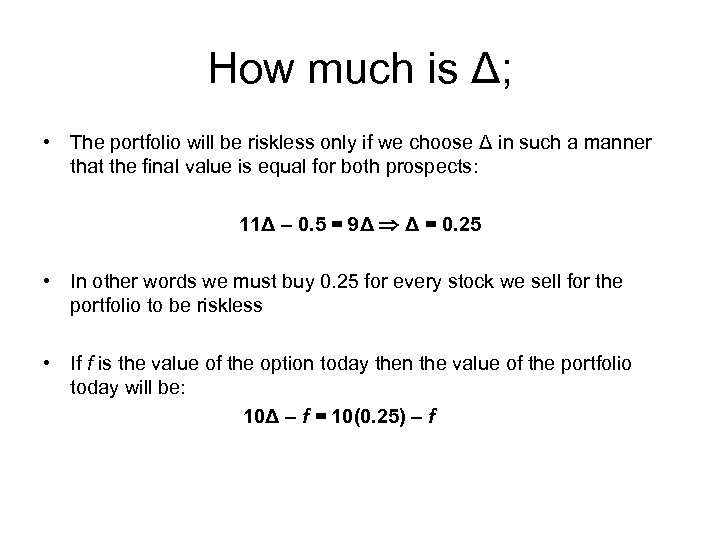How much is Δ; • The portfolio will be riskless only if we choose Δ in such a manner that the final value is equal for both prospects: 11Δ – 0. 5 = 9Δ Δ = 0. 25 • In other words we must buy 0. 25 for every stock we sell for the portfolio to be riskless • If f is the value of the option today then the value of the portfolio today will be: 10Δ – f = 10(0. 25) – f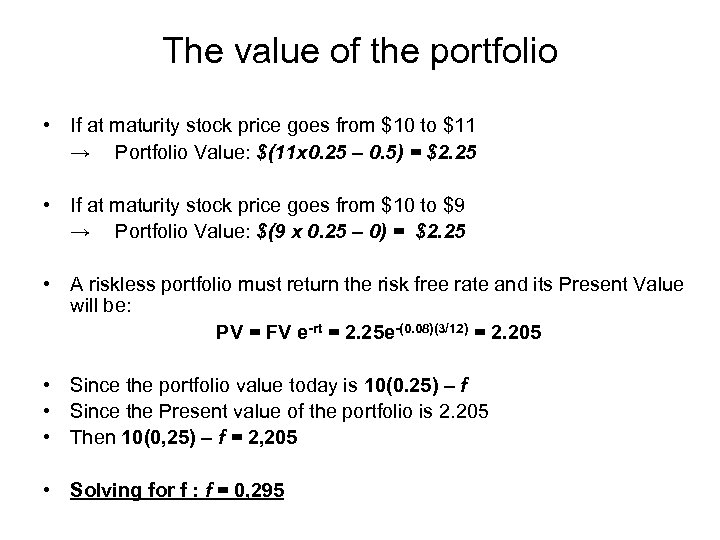The value of the portfolio • If at maturity stock price goes from \$10 to \$11 → Portfolio Value: \$(11 x 0. 25 – 0. 5) = \$2. 25 • If at maturity stock price goes from \$10 to \$9 → Portfolio Value: \$(9 x 0. 25 – 0) = \$2. 25 • A riskless portfolio must return the risk free rate and its Present Value will be: PV = FV e-rt = 2. 25 e-(0. 08)(3/12) = 2. 205 • Since the portfolio value today is 10(0. 25) – f • Since the Present value of the portfolio is 2. 205 • Then 10(0, 25) – f = 2, 205 • Solving for f : f = 0, 295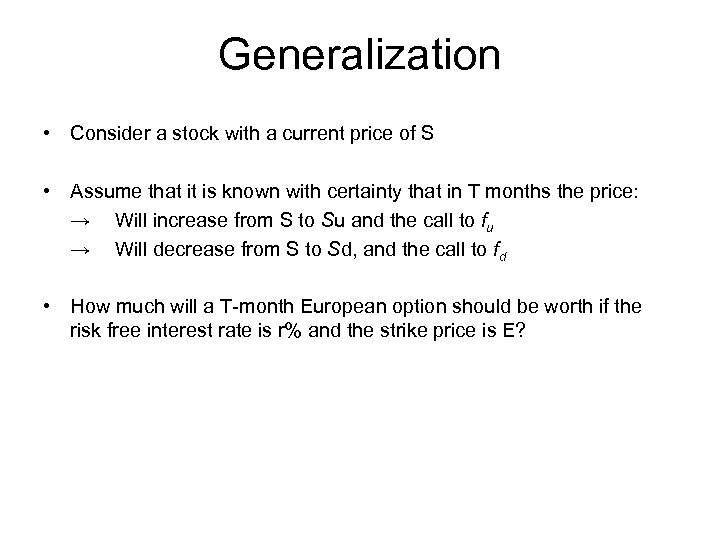Generalization • Consider a stock with a current price of S • Assume that it is known with certainty that in T months the price: → Will increase from S to Su and the call to fu → Will decrease from S to Sd, and the call to fd • How much will a T-month European option should be worth if the risk free interest rate is r% and the strike price is E?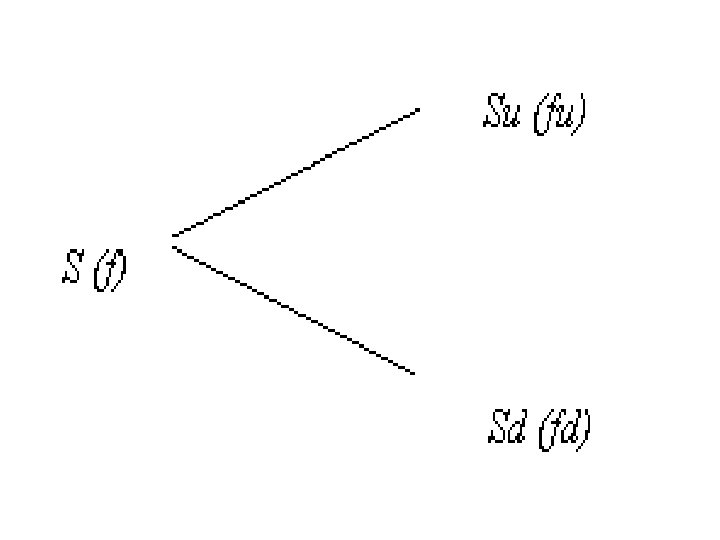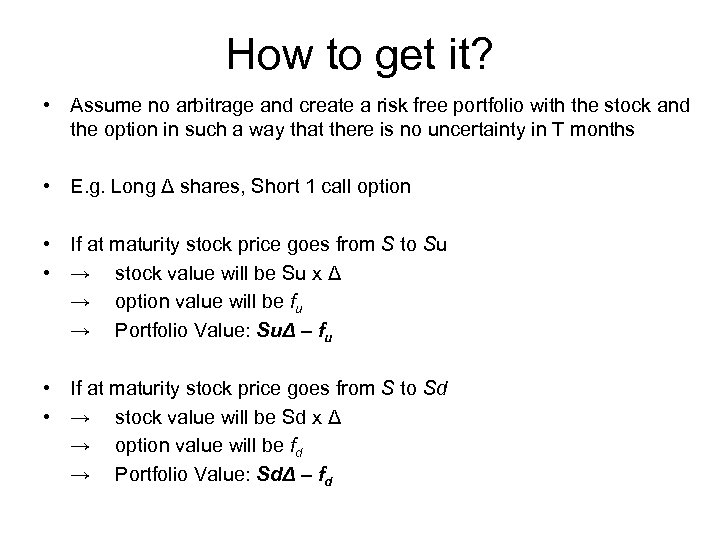How to get it? • Assume no arbitrage and create a risk free portfolio with the stock and the option in such a way that there is no uncertainty in T months • E. g. Long Δ shares, Short 1 call option • If at maturity stock price goes from S to Su • → stock value will be Su x Δ → option value will be fu → Portfolio Value: SuΔ – fu • If at maturity stock price goes from S to Sd • → stock value will be Sd x Δ → option value will be fd → Portfolio Value: SdΔ – fd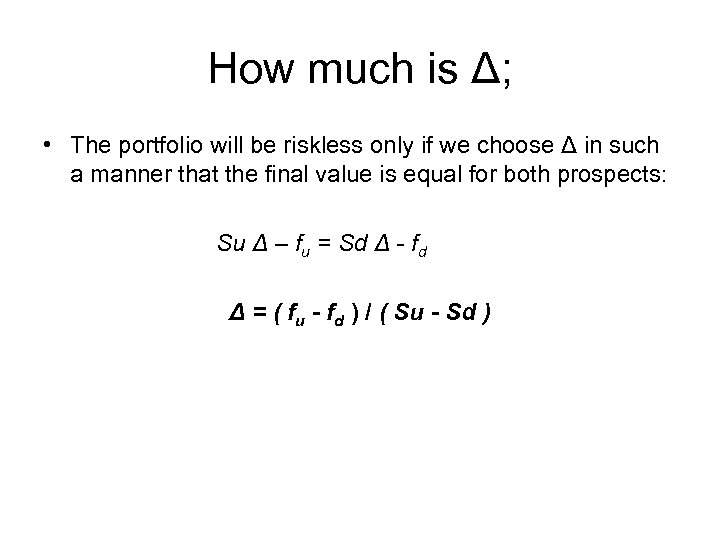How much is Δ; • The portfolio will be riskless only if we choose Δ in such a manner that the final value is equal for both prospects: Su Δ – fu = Sd Δ - fd Δ = ( fu - fd ) / ( Su - Sd )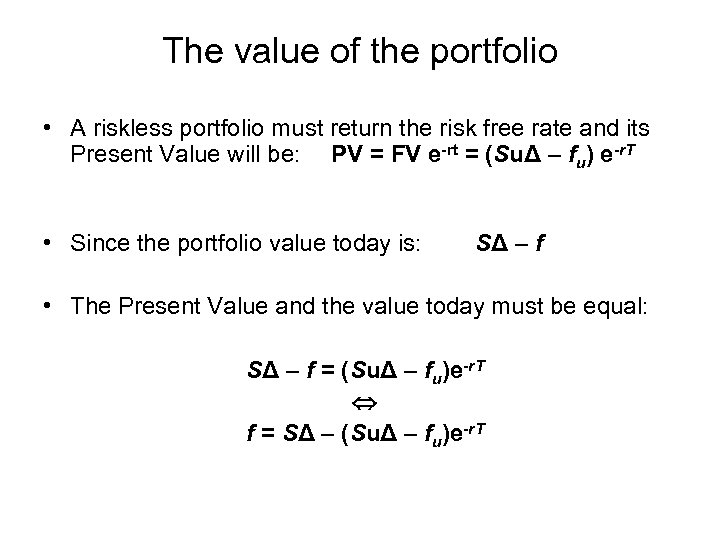The value of the portfolio • A riskless portfolio must return the risk free rate and its Present Value will be: PV = FV e-rt = (SuΔ – fu) e-r. T • Since the portfolio value today is: SΔ – f • The Present Value and the value today must be equal: SΔ – f = (SuΔ – fu)e-r. T f = SΔ – (SuΔ – fu)e-r. T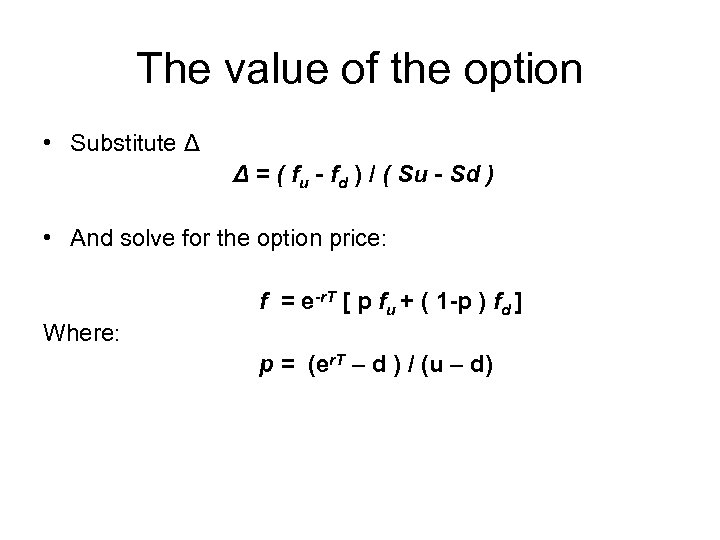The value of the option • Substitute Δ Δ = ( fu - fd ) / ( Su - Sd ) • And solve for the option price: f = e-r. T [ p fu + ( 1 -p ) fd ] Where: p = (er. T – d ) / (u – d)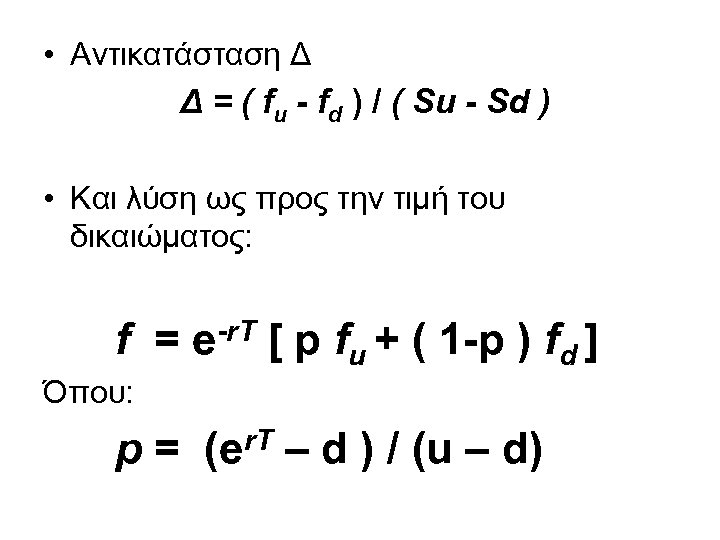• Αντικατάσταση Δ Δ = ( fu - fd ) / ( Su - Sd ) • Και λύση ως προς την τιμή του δικαιώματος: f = e-r. T [ p fu + ( 1 -p ) fd ] Όπου: p = (er. T – d ) / (u – d)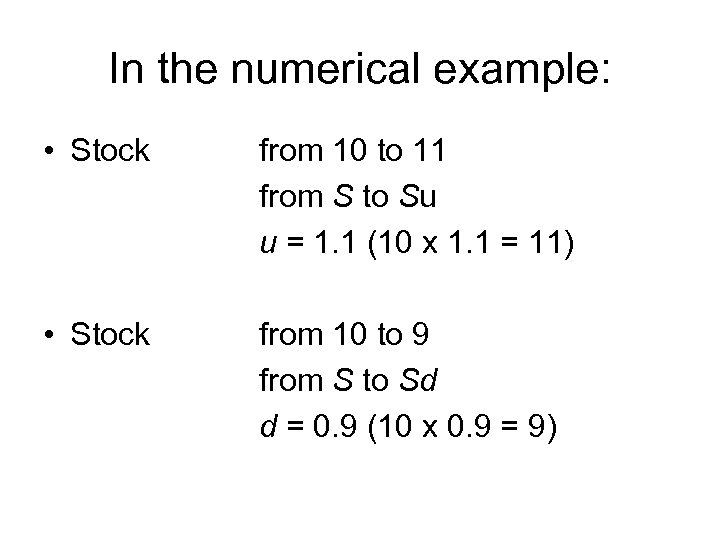In the numerical example: • Stock from 10 to 11 from S to Su u = 1. 1 (10 x 1. 1 = 11) • Stock from 10 to 9 from S to Sd d = 0. 9 (10 x 0. 9 = 9)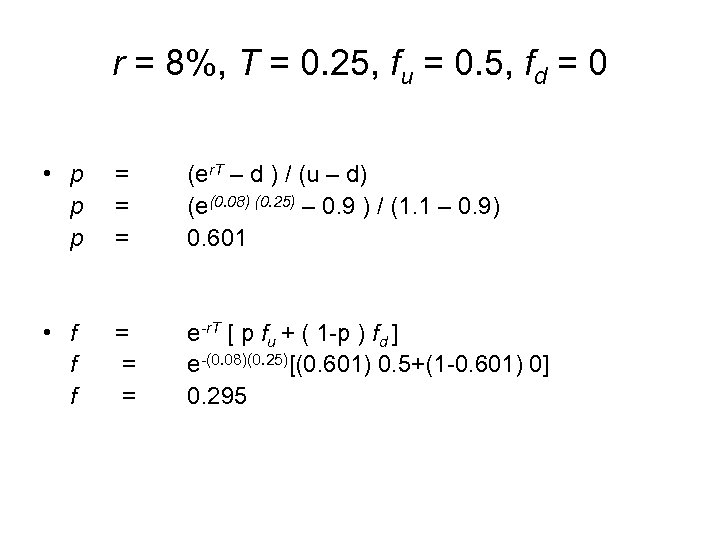r = 8%, T = 0. 25, fu = 0. 5, fd = 0 • p p p = = = (er. T – d ) / (u – d) (e(0. 08) (0. 25) – 0. 9 ) / (1. 1 – 0. 9) 0. 601 • f f f = = = e-r. T [ p fu + ( 1 -p ) fd ] e-(0. 08)(0. 25)[(0. 601) 0. 5+(1 -0. 601) 0] 0. 295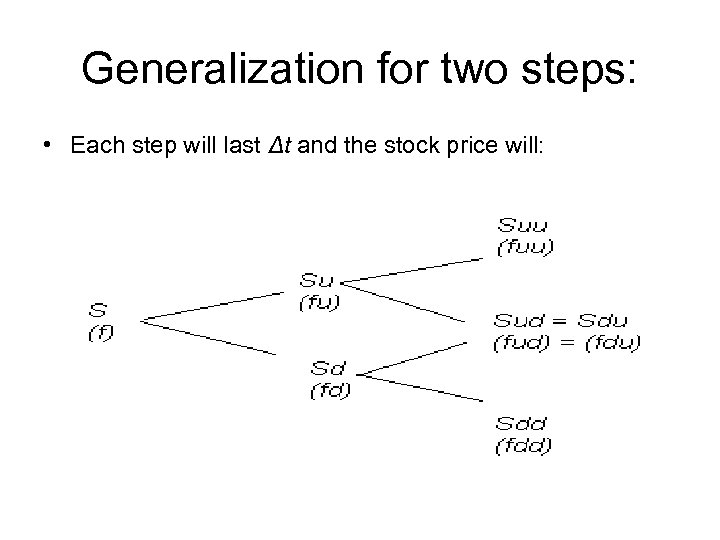Generalization for two steps: • Each step will last Δt and the stock price will: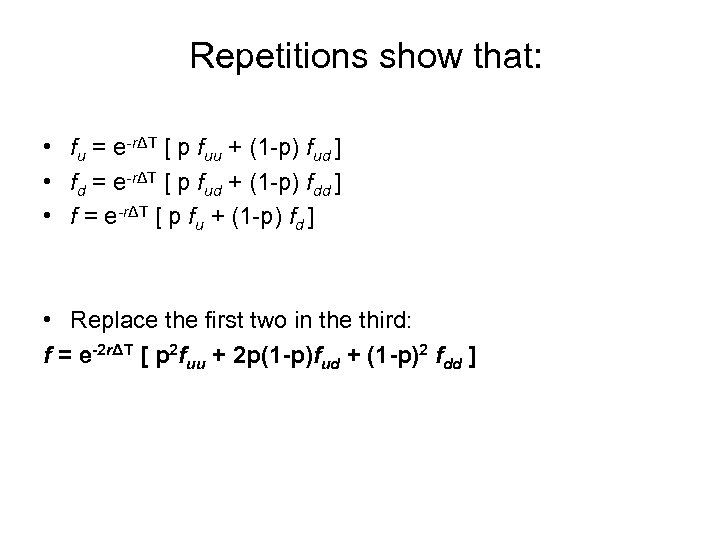Repetitions show that: • fu = e-rΔT [ p fuu + (1 -p) fud ] • fd = e-rΔT [ p fud + (1 -p) fdd ] • f = e-rΔT [ p fu + (1 -p) fd ] • Replace the first two in the third: f = e-2 rΔT [ p 2 fuu + 2 p(1 -p)fud + (1 -p)2 fdd ]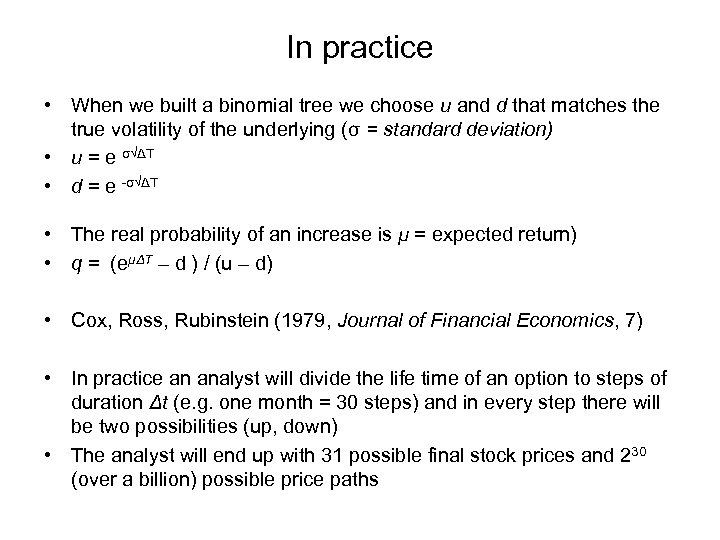In practice • When we built a binomial tree we choose u and d that matches the true volatility of the underlying (σ = standard deviation) • u = e σ√ΔΤ • d = e -σ√ΔΤ • The real probability of an increase is μ = expected return) • q = (eμΔΤ – d ) / (u – d) • Cox, Ross, Rubinstein (1979, Journal of Financial Economics, 7) • In practice an analyst will divide the life time of an option to steps of duration Δt (e. g. one month = 30 steps) and in every step there will be two possibilities (up, down) • The analyst will end up with 31 possible final stock prices and 230 (over a billion) possible price paths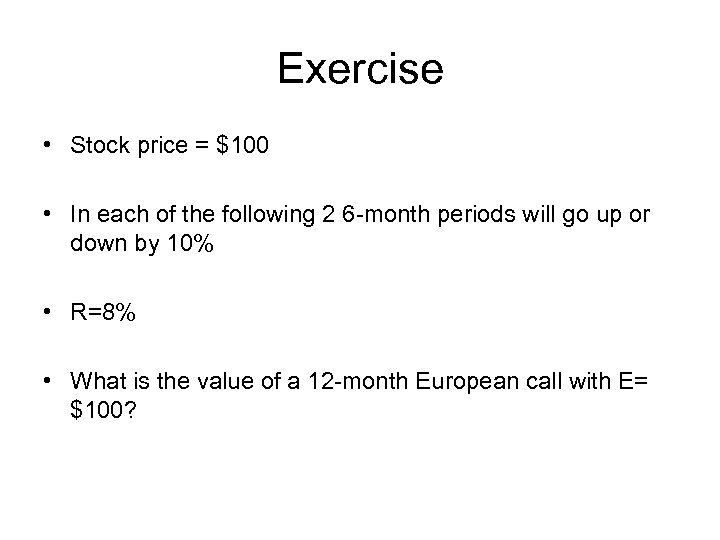Exercise • Stock price = \$100 • In each of the following 2 6 -month periods will go up or down by 10% • R=8% • What is the value of a 12 -month European call with E= \$100?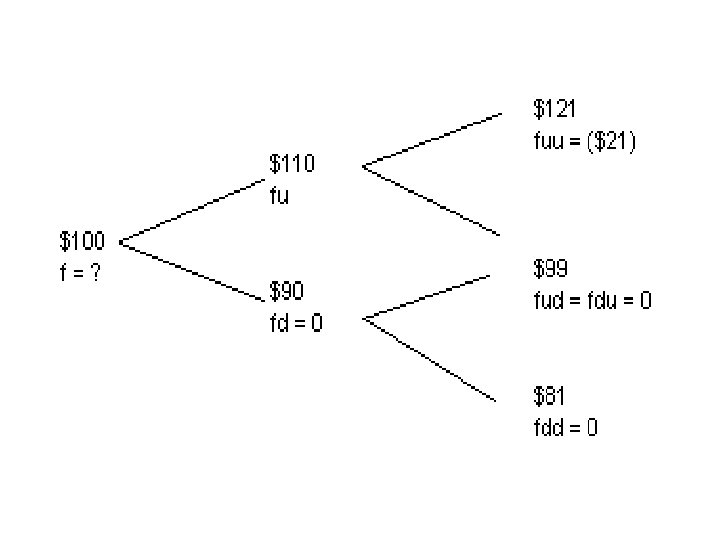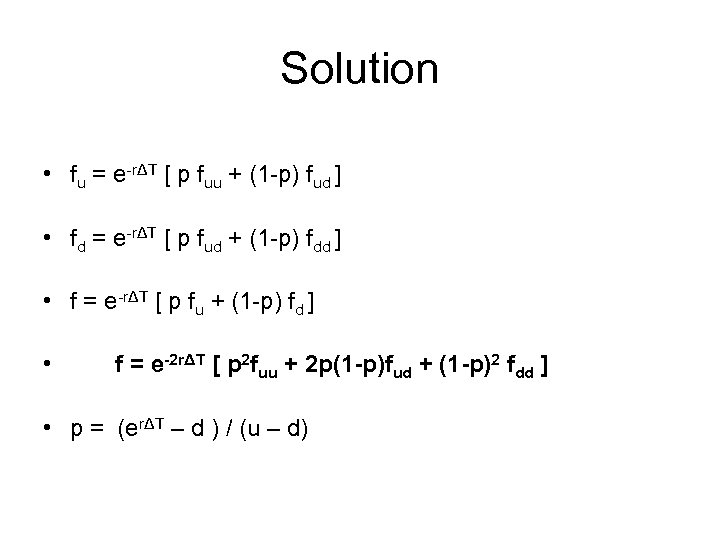Solution • fu = e-rΔT [ p fuu + (1 -p) fud ] • fd = e-rΔT [ p fud + (1 -p) fdd ] • f = e-rΔT [ p fu + (1 -p) fd ] • f = e-2 rΔT [ p 2 fuu + 2 p(1 -p)fud + (1 -p)2 fdd ] • p = (erΔT – d ) / (u – d)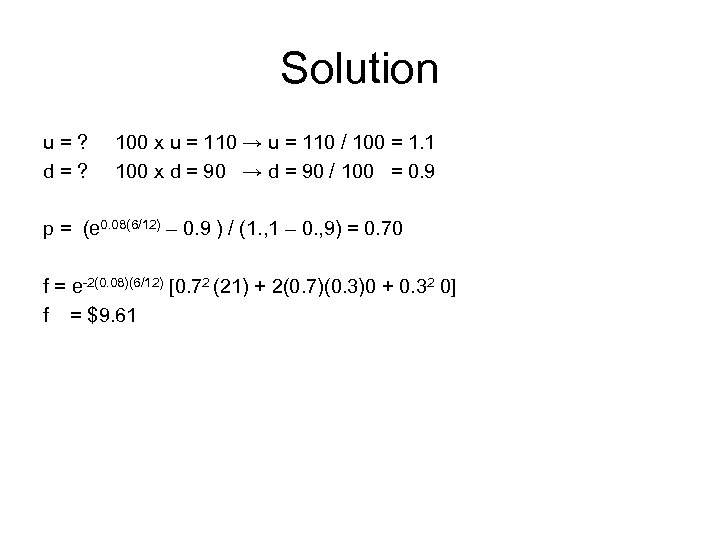Solution u=? d=? 100 x u = 110 → u = 110 / 100 = 1. 1 100 x d = 90 → d = 90 / 100 = 0. 9 p = (e 0. 08(6/12) – 0. 9 ) / (1. , 1 – 0. , 9) = 0. 70 f = e-2(0. 08)(6/12) [0. 72 (21) + 2(0. 7)(0. 3)0 + 0. 32 0] f = \$9. 61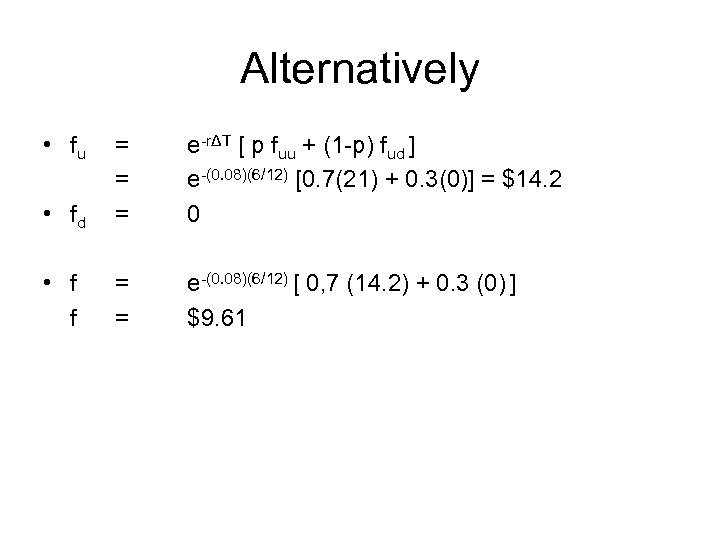Alternatively • fu • fd = = = e-rΔT [ p fuu + (1 -p) fud ] e-(0. 08)(6/12) [0. 7(21) + 0. 3(0)] = \$14. 2 0 • f f = = e-(0. 08)(6/12) [ 0, 7 (14. 2) + 0. 3 (0) ] \$9. 61